# Predicting flow rates of a pressure driven system

brunopeniche123
Hi!

I'm a student of pharmaceutical sciences which means that my physics knowledge isn’t that great ahah, if I say a big mistake in the following text, a thousand "sorrys" ahah:

So, I am now writing my master thesis and currently I am finishing writing a part where fluid mechanics are involved:

I have a pressure driven flow system, where the flow rate is controlled by a capillary, which is much smaller in terms of diameter than the rest of the tubbing, thus, having more hydraulic resistance than the rest of the system, leading to flow control. So, the flow rate is dictated by the capillary.

During my experiments measuring the flow rates, we used the Haggen-Pouiselle law to predict and change flow rate (Q= (dP. pi. r^4)/(8. viscosity. length of the capillary)). In the smallest capillary diameters (0,127mm with lengths of 150 and 60mm) flows predicted were very similar to the practical ones (flow rates were predicted using https://www.dolomite-microfluidics.com/support/microfluidic-calculator/), which made sense because the flow rate calculator uses the haggen-poiseulle law to predict flows and the flow was laminar. Perfect! :D

The problem is when I increase capillary diameter, to the 0,180 and 0,250mm. The predicted values are bigger than the real ones. Which is logical because the online calculator tells me that the flow is turbulent (although I don’t really understand that part because the value of the reynolds number given is smaller than is smaller than 2000).

So, first I was intrigued because if flow is turbulent, I should have real flows greater than the predicted, and the opposite was happening. But I forgot that part

So, I thought of the Darcy-Weisbach equation which allow us to calculate flow velocity of turbulent and laminar flows (dP = friction factor. (L/diameter). ((density.v^2)/2)). So, I searched for the friction factor of my capillary which was a PEEK capillary used in HPLC. So, in order to find the friction factor, I had to calculate the relative roughness of the capillary. I searched for the absolute roughness of PVC and other plastics and assumed a value of 0,0015 mm. I divided by the diameter of my capillary which in this calculation was the one with 0,250mm of diameter which gave me 0,006. I then used an online calculator to solve the Colebrook equation in order to have the friction factor, which gave me 0,136 (https://www.ajdesigner.com/php_colebrook/colebrook_equation.php#ajscroll) . I used the reynolds number gave by the dolomite online calculator (159.782). Making the calculations (pressure drop was 10 psi which I converted to Pa), I had a flow value of 5ml/min of flow rate (viscosity of the liquid is 1,490 cP and density is 948 kg/m^3; the liquid is THF:Water (3:2)), much higher than the real one of 2,3ml/min and the predicted of 2,9ml/min.

The first problem that I find here is that for knowing the reynold number, I have to know the velocity of the fluid, something that I wanted to predict... So, this approach doesn’t make much sense to me.

The second, is that I am getting real flow rates slower than the predictions of Haggen-Pouiselle law and it should be the opposite.

Can you help me please with this issue? :D
Is there any alternative equation to predict flow rate?
Can you see what is wrong here?

Thank you so much for your attention!

Mentor
The correct approach is to assume laminar flow, use the Hagen-Poiseulle equation, and then check to see whether the Reynolds number is less than 2000. If it is, then you're done.

Please supply some data that I can check in a more ordered fashion.

You gave no indication of the specific combination of capillary diameter and length that gave the problem results.

By any chance, is the fluid non-Newtonian?

•brunopeniche123
brunopeniche123
The correct approach is to assume laminar flow, use the Hagen-Poiseulle equation, and then check to see whether the Reynolds number is less than 2000. If it is, then you're done.

Please supply some data that I can check in a more ordered fashion.

You gave no indication of the specific combination of capillary diameter and length that gave the problem results.

By any chance, is the fluid non-Newtonian?

Good question, I really don't know but I wil search if THF:Water (3:2) is Newtonian or non Newtonion.

When I arrive home in the afternoon, I write all the data organised!

I have more data to using MeOH as a solvent which is a Newtonian fluid and it gives more or the less the same discrepancies when I increase capillary diameter. But I will re-check.

However I can write the capillaries used:
- "big red": 0.127mm diameter and 150mm length
- "small red": 0.127mm diameter and 60mm length
(In this the measurements and the predicted values using haggen-poiseuille law match really good)
- "big yellow": 0.180mm diameter and 120mm length
-"small yellow": 0.180mm diameter and 60mm length
- "blue": 0.250mm diameter and 90mm length

In this three predictions don't match becoming worse when I increase capillary diameter and decrease length. Practical flow rates are slower than the predicted ones.

Thank you :)

Last edited:
brunopeniche123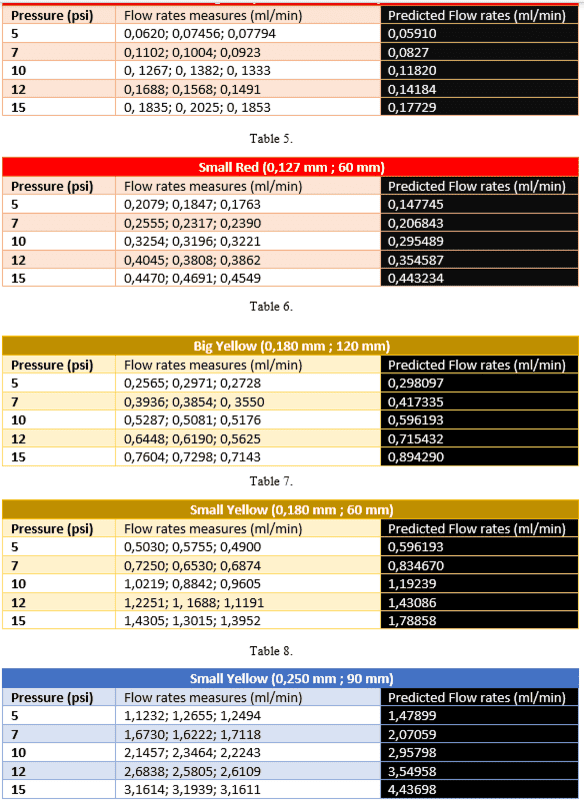Ok ,I'm back :D!

I provide the tables that I have done with the measured flow rates and the predicted ones using the Haggen-Poiseuille equation. The values with the capillary of 0,127mm and 150mm are almost a perfect match. The 0,127mm and 60mm are good too and then I starts getting worse with the values measured being smaller than the predicted (the logic would be the opposite even if the fluid is non-Newtonian, specially at higher pressures, right?)

Reynolds numbers at the highest pressure (15 psi) (viscosity of THF:Water (3:2) equals to 1,490 cP; density: 948,2 kg/m^3) of each capillary (using https://www.dolomite-microfluidics.com/support/microfluidic-calculator/):
-> 0,127mm and 150mm long capillary: Reynolds number given by the online flow calculator = 18,8522 (it says it is laminar flow)
-> 0,127mm and 60mm long capillary: Reynolds number = 47,1305 ( the calculator/software tells me that I have here turbulence, although I know that theoretical, flows are laminar until having 2000 of Reynolds number)
-> 0,180mm and 120mm long capillary: Reynolds number = 67,0932 (it says I have turbulent flow)
-> 0,180mm and 60mm long capillary: Reynolds number = 134,186 (it says I have turbulent flow)
-> 0,250mm and 90mm long capillary: Reynolds number = 239,673 (it says I have turbulent flow)

I provide also the graphs of the measured values (black graphic) and the predicted values (white one):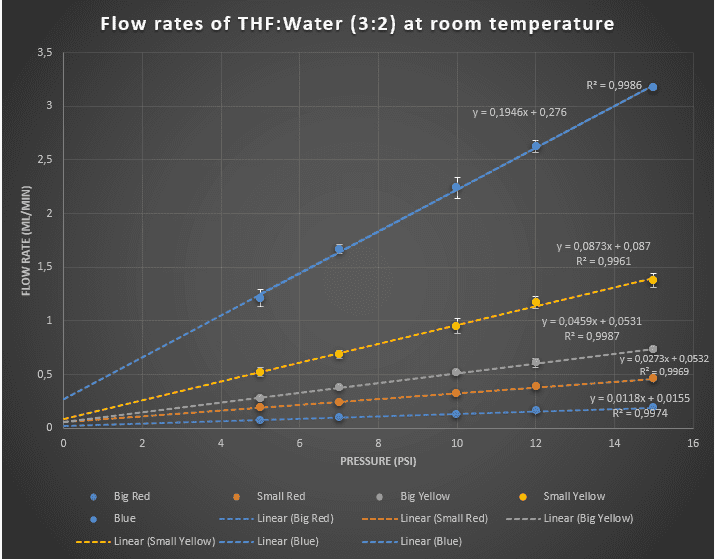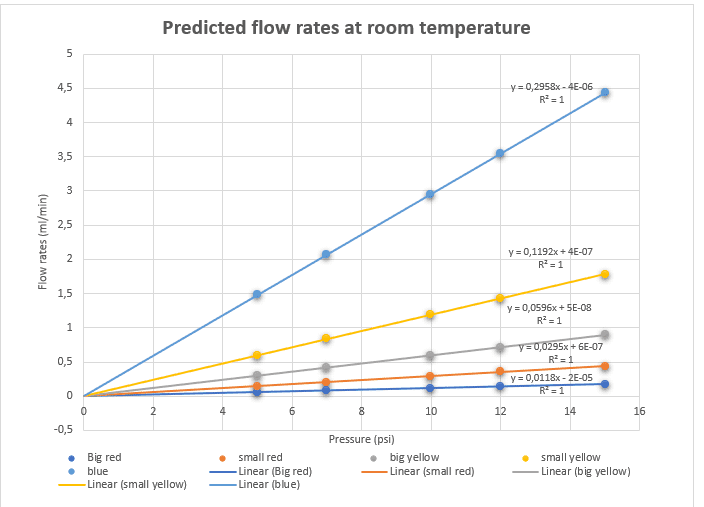So that's basically almost all the data that I have. The capillary is at the end of a pressure driven system, a mesofluidic chemical reactor is behind the capillary, due to the theory presented above, the capillary controles flow rate of all the system.

I tryed the Darcy-Weisbach equation to predict the flows that the online calculator was saying that were turbulent and were with discrepancies but I got flows a lot bigger than the real and predicted with the haggen-pouseille equation ones.

I sincerely don't know if my solvent is non-Newtonian, but I also have data from a published article by our group, with MeOH (a Newtonian fluid) and the results are the same, the flows start to don't match when we increase the capillary diameter.

Because theoretically, when the flow is turbulent, the faster the flow rate, even according to the Darcy-Weisbach equation (right?)

So, I am really in a tricky situation... What should I do? Is there another way to predict flow rates? Are you seeing what is the problem?

Thank you very much for all of your help and attention :D!

Mentor
I confirm your calculations, and, for those Reynolds numbers, the flow is obviously not turbulent. The linearity of the pressure drop vs flow rate graph confirms that the behavior is Newtonian. This leaves, in my judgment, the capillary diameters as the villain. How sure are you of the accuracy of the capillary diameters? For example, if the largest diameter were 225 instead of 250, that would remove the discrepancy there. And if the 180 was instead 168, that would remove the discrepancy there.

Last edited:
•brunopeniche123
brunopeniche123

Yeah, I think one of my colleagues measured it back in London where I did the research work. The capillaries where purchased from a company (https://www.agilent.com/en/products...upplies/capillaries-fittings/capillary-tubing) and they said that the diameter is that on their website (0,18mm and 0,25mm). However for the 0,127mm capillary, they have in their website telling its 0,12mm, so a doubt still persists.

I have sent an email to the company asking if the diameters on the website are exact.

One thing that I forgot to mention was that my pressure drive continuous flow system uses compressed air as source of pressure. I don't if it is important for that case or not.

Thank you so much again!

Mentor

Yeah, I think one of my colleagues measured it back in London where I did the research work. The capillaries where purchased from a company (https://www.agilent.com/en/products...upplies/capillaries-fittings/capillary-tubing) and they said that the diameter is that on their website (0,18mm and 0,25mm). However for the 0,127mm capillary, they have in their website telling its 0,12mm, so a doubt still persists.

I have sent an email to the company asking if the diameters on the website are exact.

One thing that I forgot to mention was that my pressure drive continuous flow system uses compressed air as source of pressure. I don't if it is important for that case or not.

Thank you so much again!
In my judgment, the flow measurements provide an accurate indirect measurement of the diameters.

Last edited:
•brunopeniche123
brunopeniche123
In my judgment, the flow measurements provide an accurate indirect measurement of the diameters.

Oh, ideed, I never thought of that. The only thing that still "annoys" me is the calculator saying that I have turbulent flows: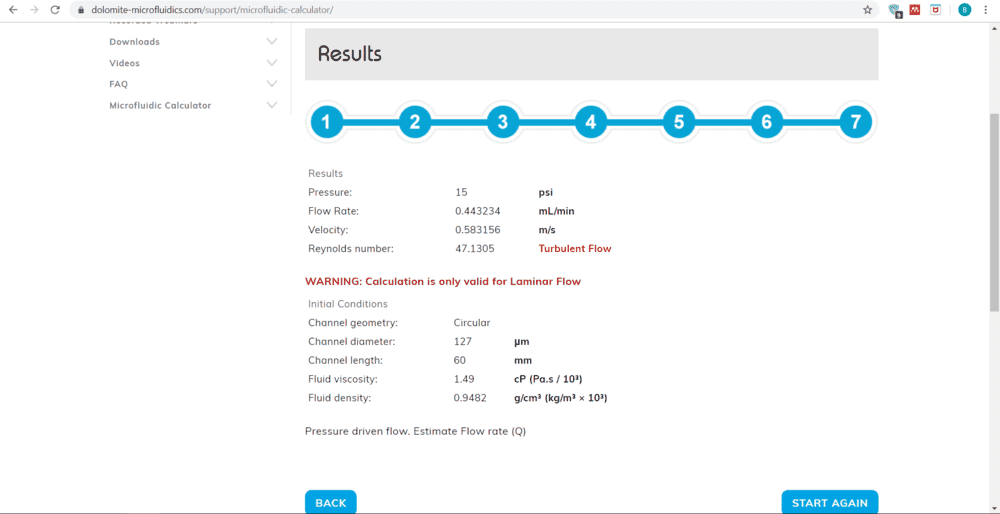But what you said indeed makes sense, or better, it is the only thing that makes sense, it explains the discrepancies.
So, I can say that this problem is solved :)
If the capillary company says something, I post here something.

Thank you so much for your help and I will for sure mention this forum and you, sir, in my thanks on my thesis! Thank you a thousand times!

brunopeniche123
I now just realized one thing... Both THF:Water and MeOH are volatile solvents. Could the evaporation that happened between the measurement and following weighting explain the discrepancies? Evaporation increases when the surface area is bigger, and I used bigger collecting flasks when the flow rates started to get close to 1ml/min. This allied with the probably not most exact measurments could explain the disprepacies.

I went to search for data with another solvent (water) and I found this: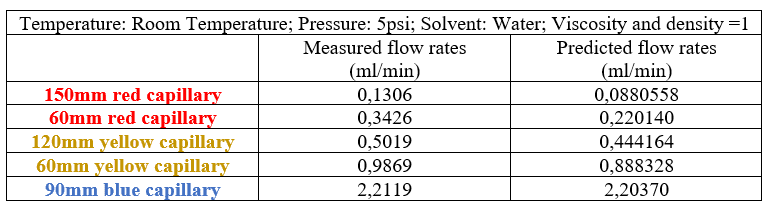It doesn't help me because know I got more accurate measurments in the bigger capillaries ahah. Oh well... ahah.
But I think this values may not be significant because I only measured once and was during my first time with the system, I could have putted the pressure not exactly at 5psi, I don't know.

So I think I'm going to stick with the logic of the diameteres of the capillaries not being exact and solvent evaporation.